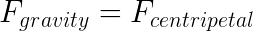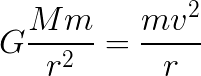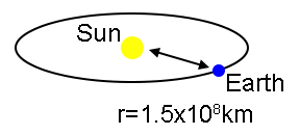# How to calculate 1+1

• Okay, so. I tried using "print(1+1)" on the Lua online demo and it's not getting me anywhere. It just returns the error "cannot print(1+1)." So, I found out how to bypass the system. I'll show you how.

So, first, we need to calculate the mass of the sun.

## Calculating the Mass of the Sun

Since the gravitational attraction of our Sun for the Earth is the centripetal force causing the Earth's circular motion around the Sun, we can use Newton's law of universal gravitation to find the mass of the Sun without visiting the Sun. This is the same technique you would use to determine the mass of Cygnus X-1 with a probe. The Earth, orbiting the Sun, plays the same role as the probe sent to orbit Cygnus X-1.

The equation is below.From that equation, it also follows this proportion:In this equation, we know G, because it's constant. Although, we need to know how far our home planet is from the Solaris and how fast mother earth is revolving around it.• The value for G is 6.67 × 10-11 N m2/kg2 (where N is Newtons)
• The distance separating the Earth and the BIG BURNING BALL OF DEATH (the orbital radius of the Earth around the Sun), r, is 1.5 × 108 km.

The Earth's velocity around the Sun is the total distance travelled over the time required for the Earth to make one complete revolution around the Sun, T.If you did everything correctly, and hopefully you did this and worked your brain a little bit, you should've gotten somewhere near 1.9891x10^30. If your answer isn't exact, don't worry, this still should work.

# Part 2: Calculating 1+1

Now that we have the mass of the Sun within our reach, as in, literally a copy-paste away, we can easily calculate 1+1 by doing the following:

`print(math.abs(1.9891e30 - 1.9891e30-2))`

AND...aw.. crap

###### (i hope you enjoyed this little joke tutorial, although this actually is how you calculate the mass of the sun. have a good day!)

• @Fifkee no u

• Make ur tutorial meaningful smh

• @Fifkee You didn't answer how to calculate 1+1

•make sure to contact nasa with this excellent knowledge, you could be making BILLIONS or even TRILLIONS with this!

Looks like your connection to Scripting Helpers was lost, please wait while we try to reconnect.